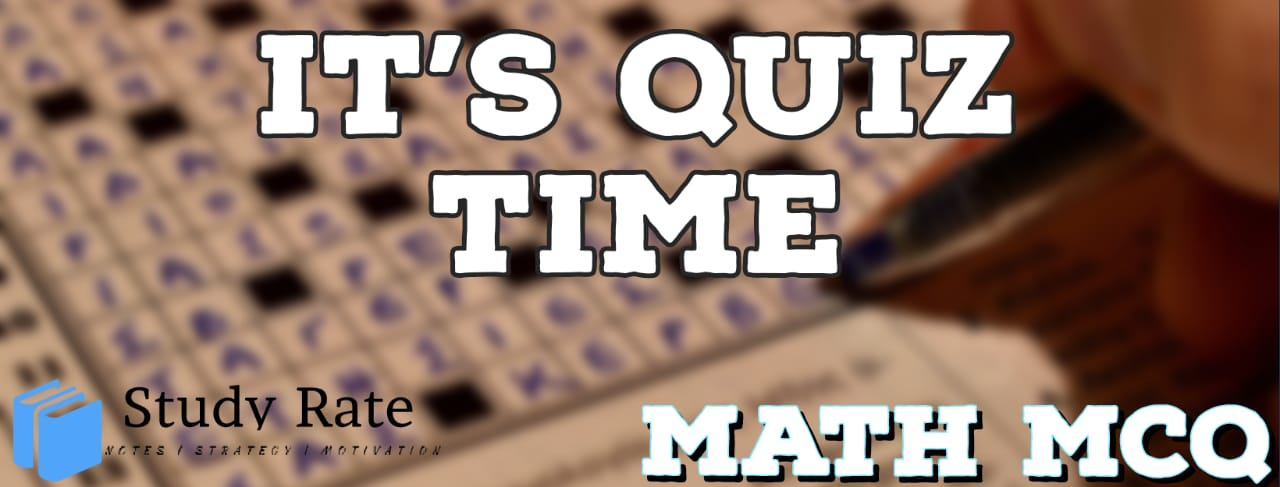## CBSE Class 10 Maths Chapter 6 – Triangles MCQ Quiz

Free PDF Download of CBSE Class 10 Maths Chapter 6 – Triangles Multiple Choice Questions with Answers. MCQ Questions for Class 10 Maths with Answers was Prepared Based on Latest Exam Pattern. Students can solve Class 10 Maths Triangles MCQs with Answers to know their preparation level

Triangles Class 10 Math MCQ Oquiz Online

1. If △ ABC ~ △ DEF such that AB = 12 cm and DE = 14 cm. Find the ratio of areas of △ ABC and △ DEF.

2. O is the point of intersection of two equal chords AB and CD such that OB = OD, then triangles OAC and ODB are

3. In the figure, PB and QA are perpendicular to segment AB. If OA = 5 cm, PO = 7cm and area (ΔQOA) = 150 cm2, find the area of ΔPOB.4. Two isosceles triangles have equal angles and their areas are in the ratio 16: 25. The ratio of corresponding heights is:

5. In triangle PQR, if PQ = 6 cm, PR = 8 cm, QS = 3 cm, and PS is the bisector of angle QPR, what is the length of QR?

6. The lengths of the diagonals of a rhombus are 16 cm and 12cm. Then, the length of the side of the rhombus is

7. A tower of height 24m casts a shadow 50m and at the same time, a girl of height 1.8m casts a shadow. Find the length of the shadow of girl.

8. If the distance between the top of two trees 20 m and 28 m tall is 17 m, then the horizontal distance between the trees is :

9. A 5 m long ladder is placed leaning towards a vertical wall such that it reaches the wall at a point 4 m high. If the foot of the ladder is moved 1.6 m towards the wall, then the distance by which the top of the ladder would slide upwards on the wall is:

10. △ ABC is such that AB = 3 cm, BC = 2 cm and CA = 2.5 cm.△ DEF is similar to △ABC.
If EF = 4 cm, then the perimeter of △DEF is –
(A) 7.5 cm
(B) 15cm
(C) 30cm
(D) 22.5cm